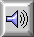LAW OF UNCERTAINTY

This law of measurement relates the determination of the FREQUENCY BAND (Df) of a sound to its duration (Dt). This relationship is most succinctly expressed by the equation:

Dt x Df = 1

meaning that the more exactly one determines either of these quantities (frequency band or time), the less exactly one knows the other, there being a close analogy here to the Heisenberg uncertainty principle in quantum physics which limits the accuracy of measuring both the position and velocity of an electron.

An example of this relationship arises when one listens via tape to fewer and fewer PERIODs of a sine tone; as the duration gets shorter, the noise content increases (i.e. Df increases). The human ear balances the perception of these two variables quite favorably, as can be concluded from the impression of a GLISSANDO as being continuous in pitch and time, or from the pitch uncertainty heard in playing a tape at twice speed.Sound Example: Short durations of a sine wave, starting at 20 ms and then reduced to 2 ms broad-band clicks, and back again.